# Proof that Hamiltonian Path is NP-Complete

Prerequisite : NP-Completeness
The class of languages for which membership can be decided quickly fall in the class of P and The class of languages for which membership can be verified quickly fall in the class of NP(stands for problem solved in Non-deterministic Turing Machine in polynomial time).
In straight words, every NP problem has its own polynomial-time verifier. A verifier for a language A is an algorithm V, where

A = {w | V accepts (w, c) for some string c}
where c is certificate or proof that w is a member of A.


We are interested in NP-Complete problems. NP-Complete problem is defined as follows:

(1)The problem itself is in NP class.
(2)All other problems in NP class can be polynomial time reducible to that.
(B is polynomial time reducible to C is denoted as)


If the 2nd condition is only satisfied then the problem is called NP-Hard.
But it is not possible to reduce every NP problem into another NP problem to show its NP-Completeness all the time. That is why if we want to show a problem is NP-Complete we just show that the problem is in NP and any NP-Complete problem is reducible to that then we are done, i.e. if B is NP-Complete andfor C in NP, then C is NP-Complete.
We have to show Hamiltonian Path is NP-Complete. Hamiltonian Path or HAMPATH in a directed graph G is a directed path that goes through each node exactly once. We Consider the problem of testing whether a directed graph contain a Hamiltonian path connecting two specified nodes, i.e.

HAMPATH = {(G, s, t) | G is directed graph with a Hamiltonian path from s to t}

To prove HAMPATH is NP-Complete we have to prove that HAMPATH is in NP. To prove HAMPATH is in NP we must have a polynomial-time verifier. Even though we don’t have a fast polynomial time algorithm to determine whether a graph contains a HAMPATH or not, if such a path is discovered somehow (maybe with exponential time brute force searching) we could easily-work it out whether the path is HAMPATH or not, in polynomial time. Here the certificate will be a Hamiltonian path from s to t itself in G if exists. So HAMPATH is in NP proved.
So, now we have to show that every problem to NP class is polynomial time reducible to HAMPATH to show its NP-Completeness. Rather we shall show 3SAT (A NP-Complete problem proved previously from SAT(Circuit Satisfiability Problem)) is polynomial time reducible to HAMPATH. We will convert a given cnf (Conjunctive Normal Form) form to a graph where gadgets (structure to simulate variables and clauses) will mimic the variables and clauses (several literals or variables connected with). We have to prove nowFor each 3-cnf formulawe will show how to build graph G with s and t, where a Hamiltonian path exists between s and t iffis satisfiable.
We start with a 3-cnf formulacontaining k clauses,where eachis a literalor. Letbe the l variables of.Now we show how to convertto a graph G. The graph G that we construct has various parts to represent the variables and clauses that appear in.
We represent each variablewith a diamond-shaped structure that contains a horizontal row of nodes as shown in following figure. We specify the number of nodes that appear in the horizontal row later.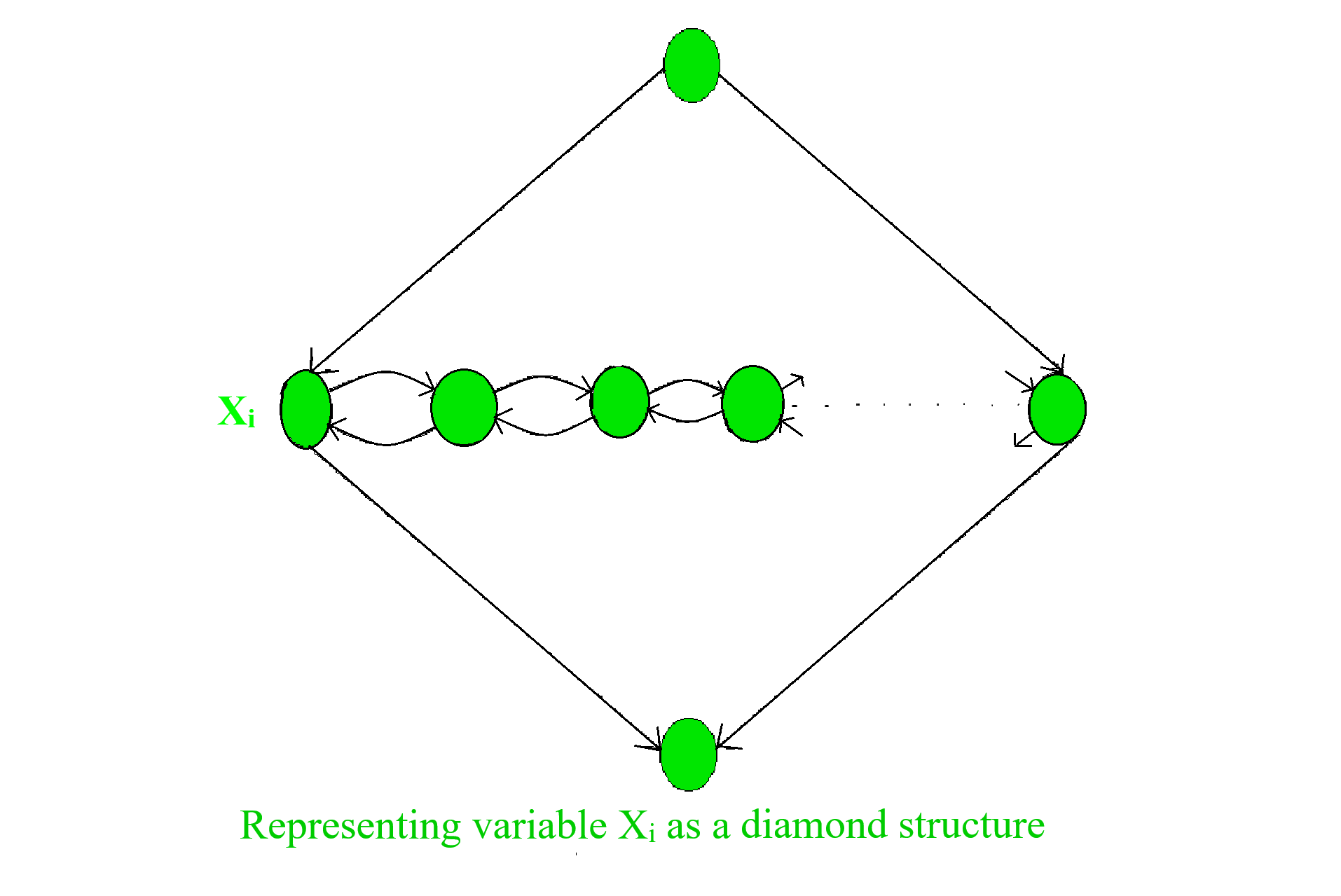The following figure depicts the global structure of G. It shows all the elements of G and their relationships, except the edges that represent the relationship of the variables to the clauses that contain them.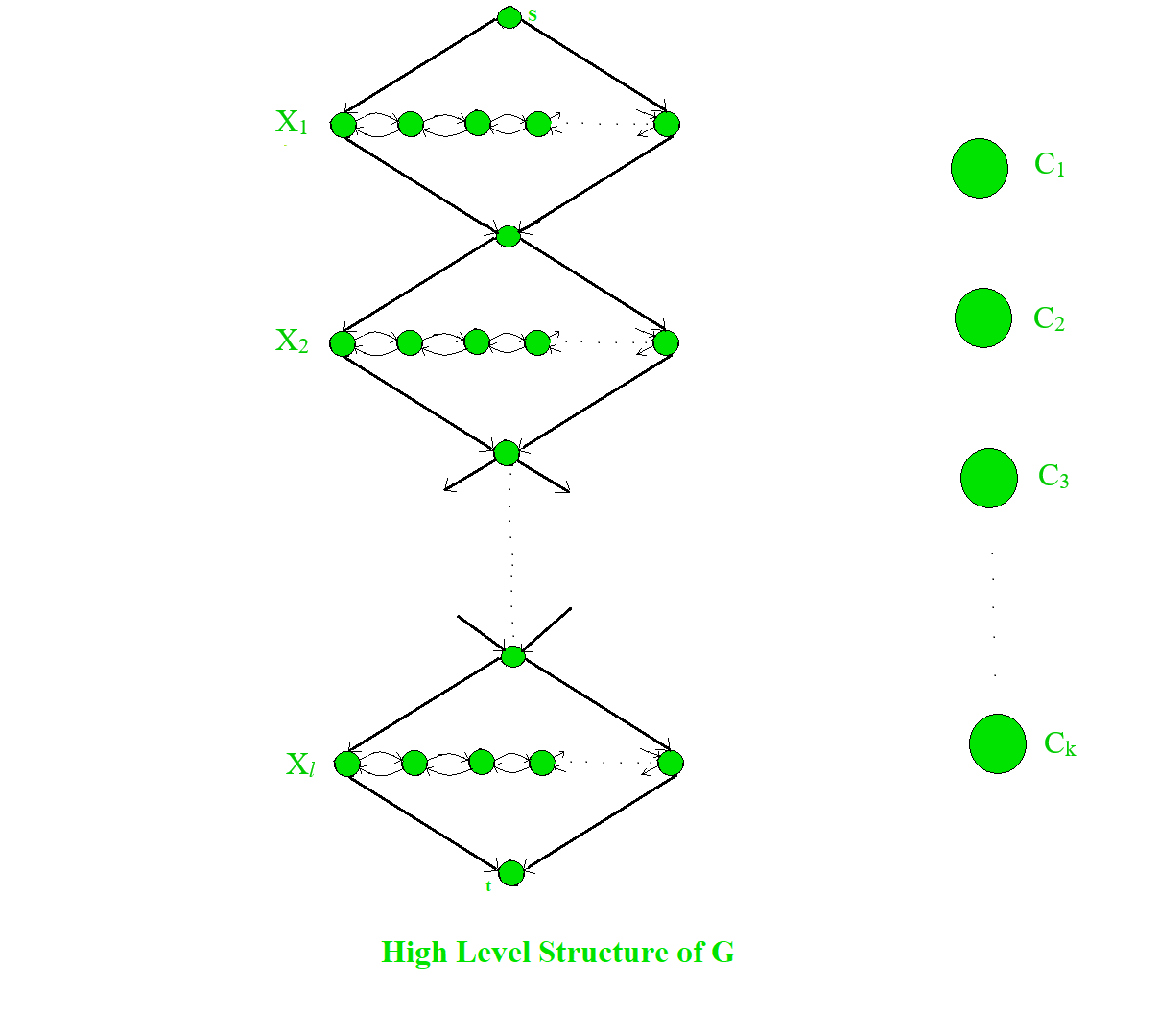Each diamond structure contains a horizontal row of nodes connected by edges running in both directions. The horizontal row contains 2k nodes (as 2 nodes for each clause) plus, k-1 extra nodes in between every two nodes for a clause plus, 2 nodes on the ends belonging to the diamonds; total 3k+1 nodes. Following diagram gives a clear picture.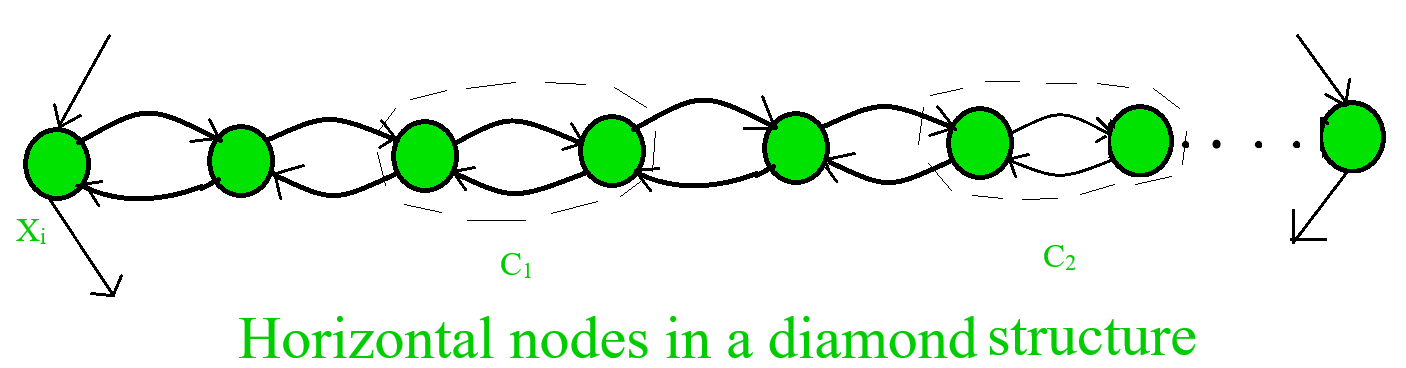If variableappears in clause, we add the following two edges from the jth pair in the ith diamoond to the jth clause node.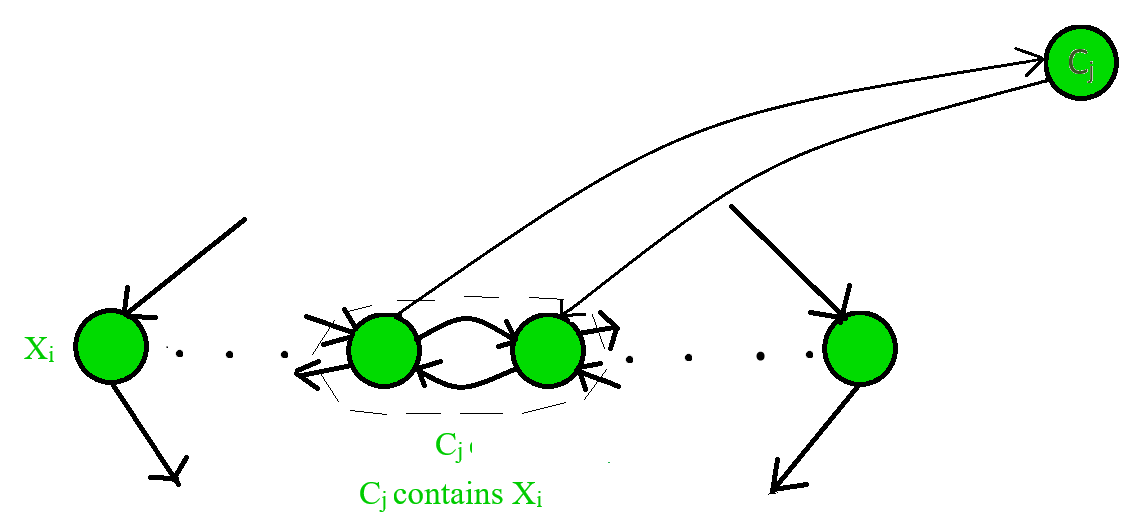Ifappears in clause c_j, we add two edges from the jth pair in the ith diamond to the jth clause node, as in the following figure.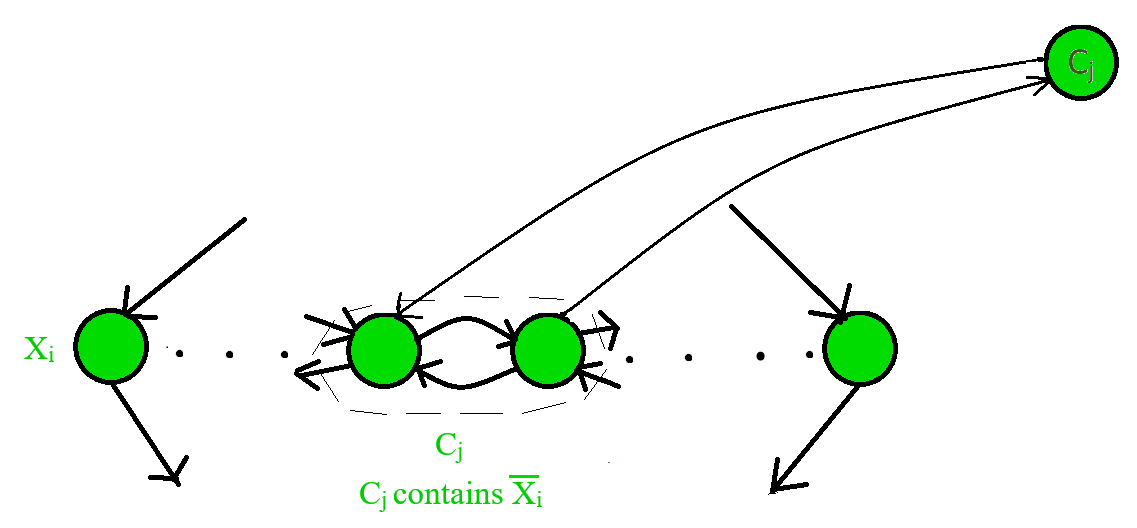After we add all the edges corresponding to each occurrence oforin each clause, the construction of G is complete. To show its correctness we will prove ifis satisfiable, a Hamiltonian path exists from s to t and conversely, if such a path existsis satisfiable.
Suppose thatis satisfiable. To demonstrate a Hamiltonian path from s to t, we first ignore the clause nodes. The path begins at s, goes through each diamond in turn and ends up at t. To hit horizontal nodes in a diamond. the path either zig-zags from left to right or zag-zigs from right to left; the satisfying assignment todetermines whetheris assigned TRUE or FALSE respectively. We show both cases in the following figure.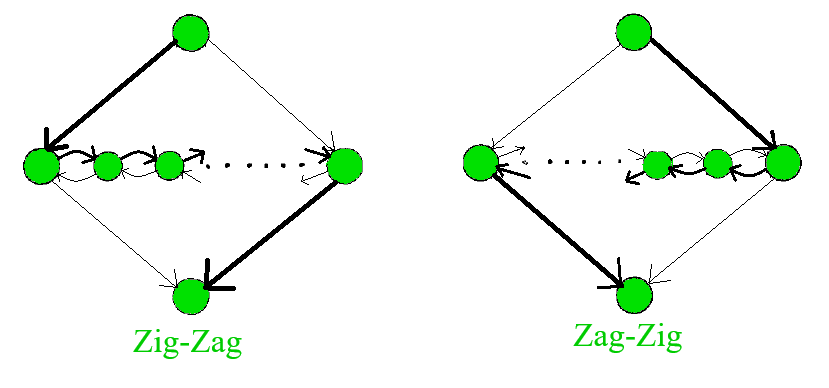So far this path covers all the nodes in G except the clause nodes. We can easily include them by adding detours at the horizontal nodes. In each clause, we select one of the literals assigned TRUE, by satisfying assignment.
If we selectedin clause, we can detour at the jth pair in the ith diamond. Doing so is possible becausemust be TRUE, so the path zig-zags from left to right through the corresponding diamond. Hence the edges to thenode are in the correct order to allow a detour and return.
Similarly, if we selectedin clause, we can detour at the jth pair in the ith diamond becausemust be FALSE, so the path zag-zigs from right to left through the corresponding diamond. Hence the edges to henode are again in the correct order to allow a detour and return. Every time one detour is taken if each literal in clause provides an option for detour. Thus each node is visited exactly once and thus Hamiltonian Path is constructed.
For the reverse direction, if G has a Hamiltonian path from s to t, we demonstrate a satisfying assignment for. If the Hamiltonian path is normal i.e. it goes through diamonds in order from top to bottom node except the detour for the closure nodes; we can easily obtain the satisfying assignment. If the path zig-zags in diamond we assign the variables as TRUE and if it zag-zigs then assign it FALSE. Because every node appears on the path by observing how the detour is taken we may determine corresponding TRUE variables.
All that remains to be shown that Hamiltonian path must be normal means the path enters a clause from one diamond but returns to another like in the following figure.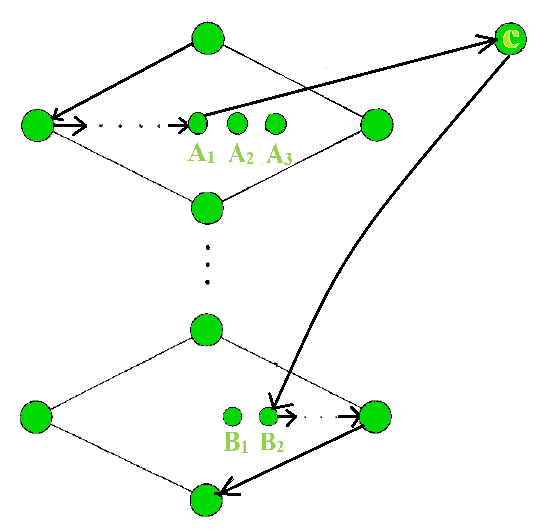The path goes from nodeto c; but instead of returning toin the same diamond, it returns toin the different diamond. If that occurs then eitherormust be a separator node. Ifwere a separator node, the only edges entering inwould be fromor. Ifwere a separator node thenandwould be in same clause, then edges that entersfrom,and c. In either case path cannot enterfrombecauseis only available node thatpoints at, so path must exitvia. Hence Hamiltonian path must be normal. This reduction obviously operates in polynomial time and hence the proof is complete that HAMPATH is NP-Complete.

Images reference: https://tr.m.wikipedia.org/wiki/Hamilton_yolu

Don’t stop now and take your learning to the next level. Learn all the important concepts of Data Structures and Algorithms with the help of the most trusted course: DSA Self Paced. Become industry ready at a student-friendly price.

My Personal Notes arrow_drop_upCheck out this Author's contributed articles.

If you like GeeksforGeeks and would like to contribute, you can also write an article using contribute.geeksforgeeks.org or mail your article to contribute@geeksforgeeks.org. See your article appearing on the GeeksforGeeks main page and help other Geeks.

Please Improve this article if you find anything incorrect by clicking on the "Improve Article" button below.